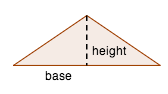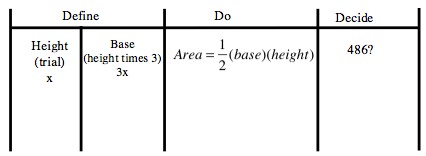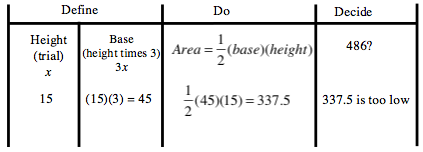### Home > CC2MN > Chapter 7 > Lesson 7.2.5 > Problem7-106

7-106.

A triangle has a base that is three times longer than its height. It has an area of $486$ sq cm.

Use the 5‑D Process to find the base and height of the triangle. Write a variable expression for each column of your table.

Describe/Draw:
The shape is a triangle and you are looking at the area.A 4 column table, first row labeled as follows: First 2 columns combined is define, third is do, fourth is decide. Row 2 labeled as follows, first: height, trial, x. Second: Base, height times 3, 3 x. Third: Area = 1 half times base times height. Fourth: 486?

If your first trial for height is $15$, what is the base?

Row 3 added as follows: 15, 15 times 3 = 45, 1 half times 45 times 15 =337.5, 337.5 is too low.Keep making trials until the correct answer is found.
Once you find the correct answer, declare it in a sentence.